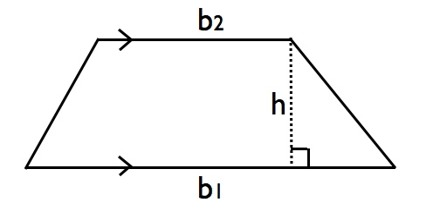Calculating the Area of a Trapezoid

A trapezoid is a quadrilateral (has 4 sides) and has only one pair of sides parallel. Area of a Trapezoid means the number of square units it takes to completely fill a trapezoid.

Formula: Average width × AltitudeHow to determine the area of a trapezoid:

• Add the lengths of the 2 parallel sides
• divide by 2 to get the average length of the parallel sides.
• Multiply this by the height (distance between the parallel sides

To find the area of a trapezoid, take the sum of its bases, multiply the sum by the height of the trapezoid, and then divide the result by 2. The formula for the area of a trapezoid is:

A = ½ (b1 + b2) × h

where b1 is base1, b2 is base2 and h is height.

Example: